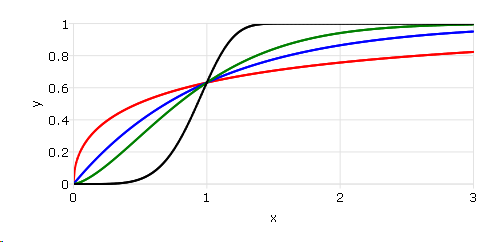# cdfWeibull¶

## Purpose¶

Computes the cumulative distribution function for the Weibull distribution.

## Format¶

p = cdfWeibull(x, shape, scale)
Parameters
• x (NxK matrix, Nx1 vector or scalar) – Values at which to evaluate the cumulative distribution function for the Weibull distribution. $$x \geq 0$$.

• shape (NxK matrix, Nx1 vector or scalar) – Shape parameter. ExE conformable with x. $$shape > 0$$.

• scale (NxK matrix, Nx1 vector or scalar) – Scale parameter, ExE conformable with x. $$scale > 0$$.

Returns

p (NxK matrix, Nx1 vector or scalar) – Each element in p is the cumulative distribution function of the Weibull distribution evaluated at the corresponding element in x.

## Examples¶

Calculate the cdf for the Weibull distribution with different shape parameters.

// Values
x = seqa(0,0.01,301);

// Shape parameter
shape = 0.5~1~1.5~5;

// Scale parameter
scale = 1;

p = cdfWeibull(x, shape, scale);
plotxy(x, p);


After running above code,## Remarks¶

The Weibull cumulative distribution function is defined as:

$f(x; k, \lambda) = 1 - e^{-(x/\lambda)k}$